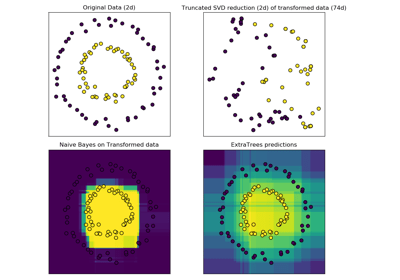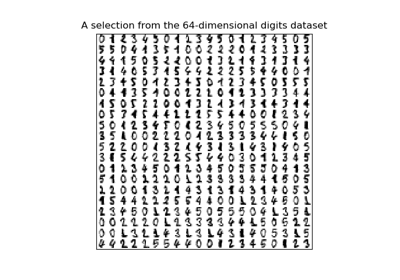/scikit-learn

# sklearn.decomposition.TruncatedSVD

`class sklearn.decomposition.TruncatedSVD(n_components=2, algorithm=’randomized’, n_iter=5, random_state=None, tol=0.0)` [source]

Dimensionality reduction using truncated SVD (aka LSA).

This transformer performs linear dimensionality reduction by means of truncated singular value decomposition (SVD). Contrary to PCA, this estimator does not center the data before computing the singular value decomposition. This means it can work with scipy.sparse matrices efficiently.

In particular, truncated SVD works on term count/tf-idf matrices as returned by the vectorizers in sklearn.feature_extraction.text. In that context, it is known as latent semantic analysis (LSA).

This estimator supports two algorithms: a fast randomized SVD solver, and a “naive” algorithm that uses ARPACK as an eigensolver on (X * X.T) or (X.T * X), whichever is more efficient.

Read more in the User Guide.

Parameters: `n_components : int, default = 2` Desired dimensionality of output data. Must be strictly less than the number of features. The default value is useful for visualisation. For LSA, a value of 100 is recommended. `algorithm : string, default = “randomized”` SVD solver to use. Either “arpack” for the ARPACK wrapper in SciPy (scipy.sparse.linalg.svds), or “randomized” for the randomized algorithm due to Halko (2009). `n_iter : int, optional (default 5)` Number of iterations for randomized SVD solver. Not used by ARPACK. The default is larger than the default in `randomized_svd` to handle sparse matrices that may have large slowly decaying spectrum. `random_state : int, RandomState instance or None, optional, default = None` If int, random_state is the seed used by the random number generator; If RandomState instance, random_state is the random number generator; If None, the random number generator is the RandomState instance used by `np.random`. `tol : float, optional` Tolerance for ARPACK. 0 means machine precision. Ignored by randomized SVD solver. `components_ : array, shape (n_components, n_features)` `explained_variance_ : array, shape (n_components,)` The variance of the training samples transformed by a projection to each component. `explained_variance_ratio_ : array, shape (n_components,)` Percentage of variance explained by each of the selected components. `singular_values_ : array, shape (n_components,)` The singular values corresponding to each of the selected components. The singular values are equal to the 2-norms of the `n_components` variables in the lower-dimensional space.

#### Notes

SVD suffers from a problem called “sign indeterminacy”, which means the sign of the `components_` and the output from transform depend on the algorithm and random state. To work around this, fit instances of this class to data once, then keep the instance around to do transformations.

#### References

Finding structure with randomness: Stochastic algorithms for constructing approximate matrix decompositions Halko, et al., 2009 (arXiv:909) http://arxiv.org/pdf/0909.4061

#### Examples

```>>> from sklearn.decomposition import TruncatedSVD
>>> from sklearn.random_projection import sparse_random_matrix
>>> X = sparse_random_matrix(100, 100, density=0.01, random_state=42)
>>> svd = TruncatedSVD(n_components=5, n_iter=7, random_state=42)
>>> svd.fit(X)
TruncatedSVD(algorithm='randomized', n_components=5, n_iter=7,
random_state=42, tol=0.0)
>>> print(svd.explained_variance_ratio_)
[0.0606... 0.0584... 0.0497... 0.0434... 0.0372...]
>>> print(svd.explained_variance_ratio_.sum())
0.249...
>>> print(svd.singular_values_)
[2.5841... 2.5245... 2.3201... 2.1753... 2.0443...]
```

#### Methods

 `fit`(X[, y]) Fit LSI model on training data X. `fit_transform`(X[, y]) Fit LSI model to X and perform dimensionality reduction on X. `get_params`([deep]) Get parameters for this estimator. `inverse_transform`(X) Transform X back to its original space. `set_params`(**params) Set the parameters of this estimator. `transform`(X) Perform dimensionality reduction on X.
`__init__(n_components=2, algorithm=’randomized’, n_iter=5, random_state=None, tol=0.0)` [source]
`fit(X, y=None)` [source]

Fit LSI model on training data X.

Parameters: `X : {array-like, sparse matrix}, shape (n_samples, n_features)` Training data. `y : Ignored` `self : object` Returns the transformer object.
`fit_transform(X, y=None)` [source]

Fit LSI model to X and perform dimensionality reduction on X.

Parameters: `X : {array-like, sparse matrix}, shape (n_samples, n_features)` Training data. `y : Ignored` `X_new : array, shape (n_samples, n_components)` Reduced version of X. This will always be a dense array.
`get_params(deep=True)` [source]

Get parameters for this estimator.

Parameters: `deep : boolean, optional` If True, will return the parameters for this estimator and contained subobjects that are estimators. `params : mapping of string to any` Parameter names mapped to their values.
`inverse_transform(X)` [source]

Transform X back to its original space.

Returns an array X_original whose transform would be X.

Parameters: `X : array-like, shape (n_samples, n_components)` New data. `X_original : array, shape (n_samples, n_features)` Note that this is always a dense array.
`set_params(**params)` [source]

Set the parameters of this estimator.

The method works on simple estimators as well as on nested objects (such as pipelines). The latter have parameters of the form `<component>__<parameter>` so that it’s possible to update each component of a nested object.

Returns: self
`transform(X)` [source]

Perform dimensionality reduction on X.

Parameters: `X : {array-like, sparse matrix}, shape (n_samples, n_features)` New data. `X_new : array, shape (n_samples, n_components)` Reduced version of X. This will always be a dense array.

## Examples using `sklearn.decomposition.TruncatedSVD`Column Transformer with Heterogeneous Data SourcesHashing feature transformation using Totally Random TreesManifold learning on handwritten digits: Locally Linear Embedding, Isomap…Clustering text documents using k-means